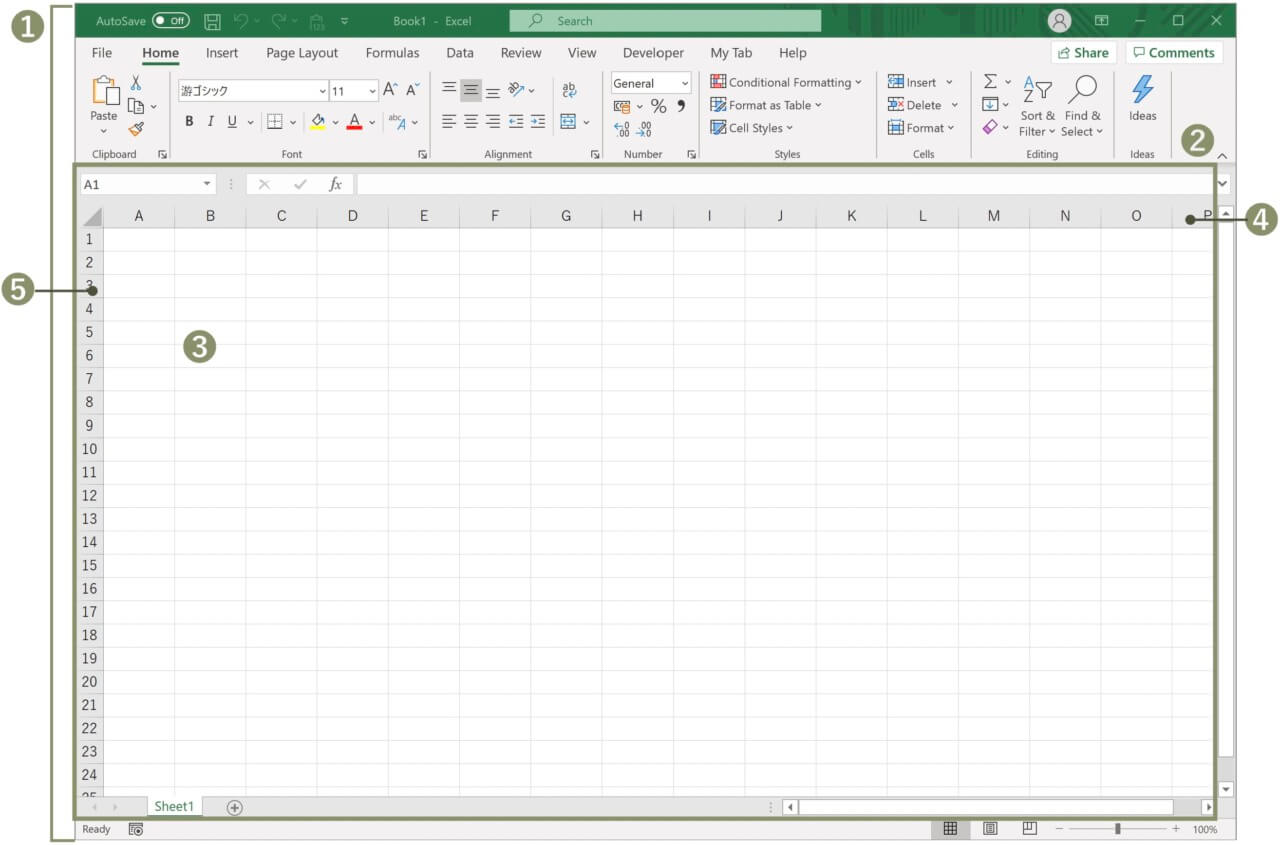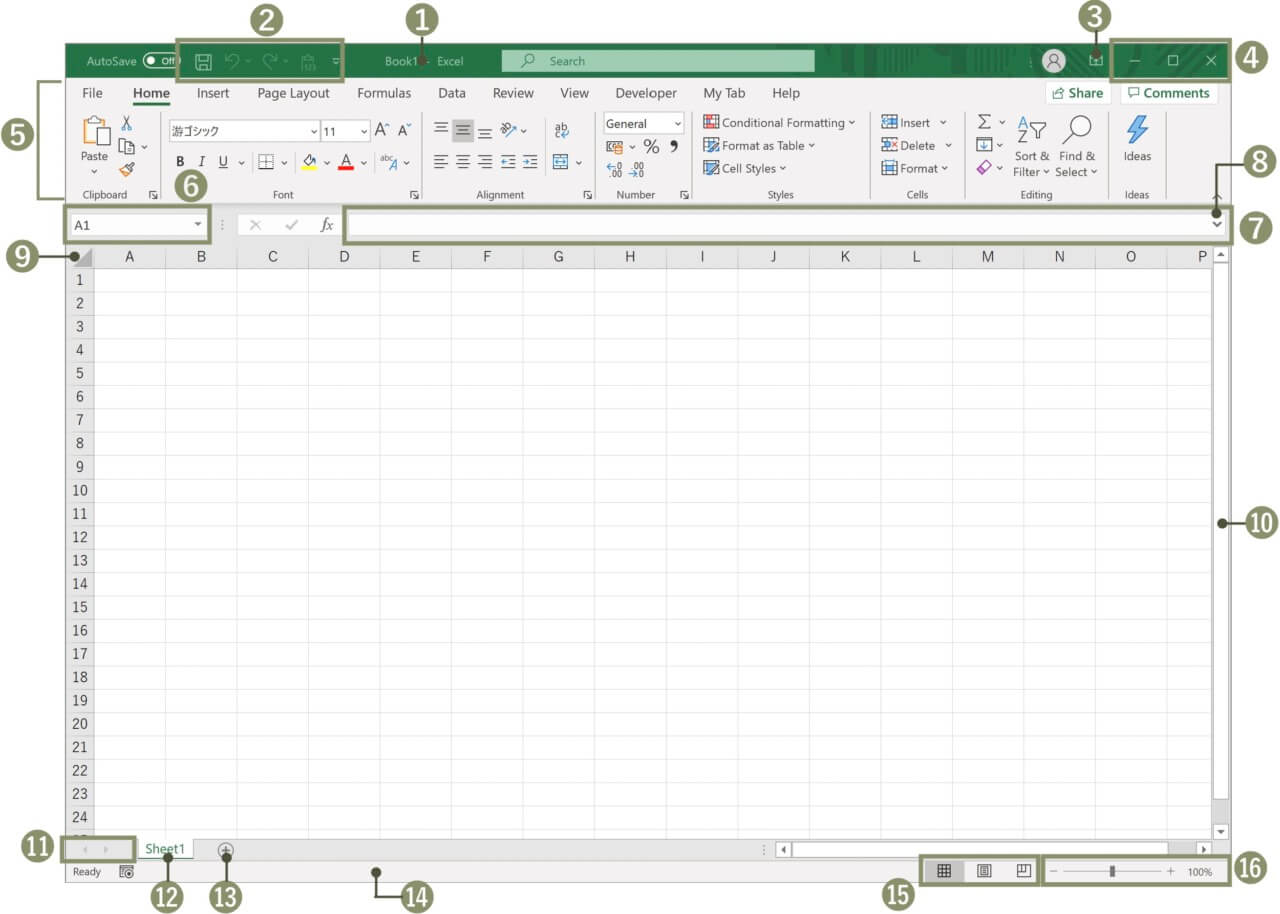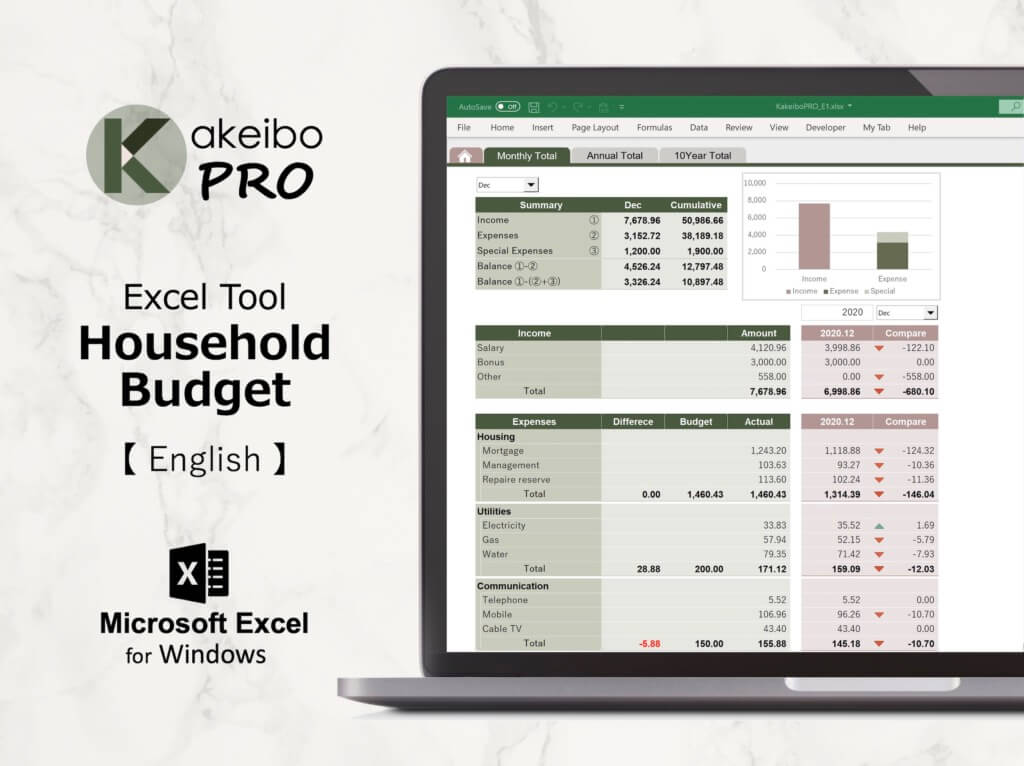MS EXCEL

## Basic knowledge of Excel

Excel is a spreadsheet application developed by Microsoft in the United States. You can enter numbers in cells and have them calculated, create a table, or graph it. If you can master it, it will be very useful for creating documents and summarizing data at work and at home.

In this lesson, you can learn the basics of Excel: Excel overview, Basic elements, and Screen structure.

##### Contents

Apprication: Microsoft Excel 2019 (Office 365)

1

## Excel Overview

Excel is a spreadsheet software that has various functions such as calculation, table creation, and graph creation based on entered data. The main features of Excel are as follows.

##### 1.　Calculation

By entering a formula, you can calculate the values entered in the cells. If you change the data, it is automatically recalculated. There are a variety of functions for calculations, you can do advanced calculations instantly by using a combination of functions.

##### 2. Table creation

It provides a variety of features for creating tables, so you can create tables that are easy to see and presentable.

##### 3. Graph creation

You can easily create graphs based on the data you have entered. You can create various types of graphs such as bar charts, pie charts, line charts and scatter plots.

##### 4. Data management

You can sort the entered data or extract specific data. It provides useful features for managing large amounts of data and can be used as a database.

##### 5. Work Automation

Excel has a feature called “Record Macro”. You can record a series of operations as a macro and execute the recorded series of operations. By recording frequently occurring operations as macros, you can work more efficiently without repeating the same operations.

2

## Excel basic elements

Here are the basic elements of Excel.##### 1. Book

In Excel, a file is called a “book”. You can open multiple books and switch between windows as you work, and the window that is being processed is called the “active window”.

##### 2. Sheet

The area where you create tables and graphs is called a “worksheet” or “sheet”. A book has one sheet and you can insert or delete sheets. The sheet that is being processed is called the “active sheet”.

##### 3. Cell

Each column and row that intersects is called a “cell”. The position is indicated by the column number and the row number, and the cell in the 5th row of column A is “A5”. The cell that is being processed is called the “active cell”.

It indicates the column number of the sheet. There are 16,384 columns from column [A] to column [XFD].

It indicates the row number of the sheet. There are 1,048,576 rows from row  to row .

3

## Excel screen structure

You can learn about the names and roles of the various parts of the Excel screen.##### 1. Title Bar

The name of file and application are displayed.

You can register frequently used commands. By default, three commands are registered: Save, Undo and Redo.

##### 3. Ribbon Display Options

You can hide or show the ribbon.

##### 4. Window buttons

[Minimize] The window is temporarily hidden and appears as an icon in the taskbar.
[Maximize] The window is maximized to fill the screen.※
[Close] You can close Excel.
※When the window is maximized, the button switches from [Maximize] to [Restore].

##### 5. Ribbon

You can use ribbon to execute a command. They are grouped into tabs for each relevant function.

##### 6. Name Box

It displays the position of the active cell.

##### 7. Formula Bar

It displays the content of the active cell.

##### 8. Expand Formula Bar

You can expand the formula bar to expand the display area.

##### 9. Select All Button

You can select all cells in the sheet.

##### 10. Scroll Bar

You can move the display area of the sheet.

##### 11. Sheet tab scroll buttons

You can move the display area of the sheet tab.

##### 12. Worksheet Tab

It is a tab to identify the sheet.

##### 13. Insert Worksheet Button

You can add more sheets as needed.

##### 14. Status Bar

It displays the current work status and processing steps.

##### 15. Views

You can change the view by choosing [Normal], [Page Layout] or [Page Bread Preview]

##### 16. Zoom Slider

You can drag the zoom slider to change the zoom level.

### PICKUP PRODUCTKakeibo makes budgeting, payment management, and household budget analysis easy! What does this tool provide you?